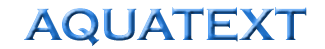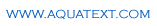The Free On-line Aquaculture DictionaryBath Treatment Calculation Example
 Example  - Formalin @ 200ppm to a tank 7.5m diameter and 2m deep Calculate the volume of water in the tank: Area = 3.1415 x radius2 x depth = 3.1415 x (7.5/2)2 x 2 = 88.35m3 = 88,350,000 millilitres Volume of chemical required = (volume ml / 1000000) x concentration (ppm or mg/l) = (88,350,000 / 1,000,000) x 200 = 17,670 millilitres formalin Therefore to dose the tank with 200ppm of formalin requires 17,670 ml (or 17.67 litres) of formalin. Note that the above calculation also works for chemicals dosed by weight as mg/l. 1 ml should be taken as the equivalent of 1 mg.